# How to lookup cells having certain text and returns the Certain Text in Excel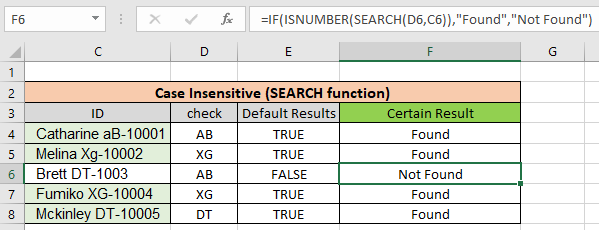In this article, we will learn How to look up cells having certain text and return the Certain Text in Excel.

Scenario :

Identify particular text in a cell or different word in given cells. For example finding the department ID from a database. I think you must have thought to do it manually but time constraint. You are here at the right place to learn How to check if a cell contains specific text.

Generic formula:

The text you are looking for can either be exact or case insensitive. Case insensitive means formula looks for AG can return ag, Ag, AG or aG.

Case insensitive formula:

 =ISNUMBER(SEARCH(find_text,within_text)

find_text : text to find

within_text : to find in text

Case sensitive formula:

 =ISNUMBER(FIND(find_text,within_text)

find_text : text to find

within_text : to find in text

Note:

The above formulas will return True or False. Use the IF function with formula to return YES or NO. Use the below formula to get the certain required result format.

Case insensitive formula:

 =IF(ISNUMBER(SEARCH(find_text,within_text)),"value_if_true",value_if_false)

find_text : text to find

within_text : to find in text

Case sensitive formula:

 =IF(ISNUMBER(FIND(find_text,within_text)),"value_if_true",value_if_false)

find_text : text to find

within_text : to find in text

Note: In The above mentioned formula you can input values like YES/NO or Found/Notfound.

Example :

All of these might be confusing to understand. Let's understand how to use the function using an example. Here we have some employees to look up by the given department Id. Employee id has name, department id and particular id. Here lookup text is in column D and within text is in Column C.

Use the formula:

 =ISNUMBER(SEARCH(D4,C4))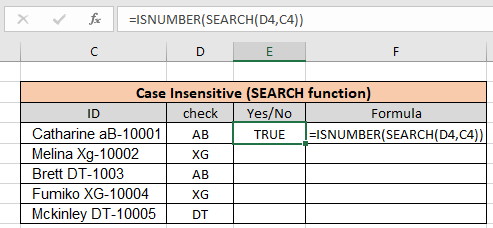As you can see the formula finds aB when you looked for

AB using the Search function. Copy the formula to the rest of the cells using the Ctrl + D or dragging it down from the right bottom (tiny box) of the applied cell.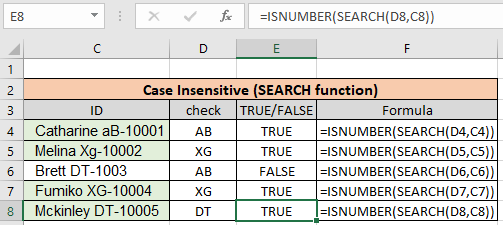As you can see we found all the given department id employees using the above method. Now we will check if all cells contain specific text.

Another Example: (Case Sensitive)

Here we have been given a list of Employee Id information in  column A and the look up department is "XG". Here we need to find the department "XG" in all cells. "XG" must be exact as there are two different departments that go by "Xg". So check only "XG" in all cells and return "Yes" if found and return "No" If not.

Use the formula:

 =ISNUMBER(FIND(D4,C4))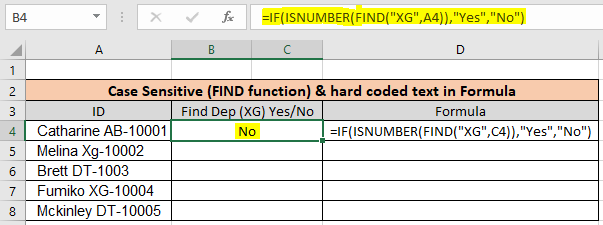As you can see First employee doesn't belong to "XG" so the formula returns "No" using the FIND function. Copy the formula to the rest of the cells using the Ctrl + D or dragging it down from the right bottom (tiny box) of the applied cell.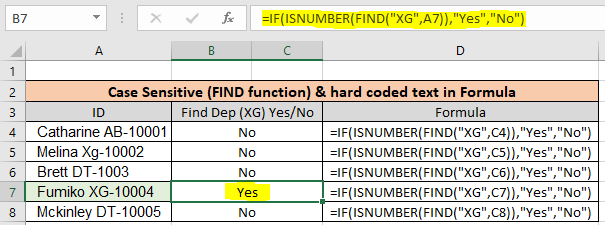As you can see there is only one employee in "XG" department. This formula is useful wherever the database contains multiple information of a row in one cell.

Check if cell Matches multiple text

In the above example we lookup one given text in cells. If we have multiple texts then we use the SUMPRODUCT function, This formula returns TRUE/FALSE as per the value found/ Not found.

Use the formula:

 =SUMPRODUCT(--ISNUMBER(SEARCH(,A1)))>0

Here are all the observational notes using the above explained formulas in Excel
Notes :

1. Use the "find_text" in quotes when using hard coded values. Or else use cell reference as explained in the first example.
2. SEARCH is case insensitive function whereas FIND is the case Sensitive dunction.
3. Use the IF function, if you want to get the result in required forms like YES/NO or Found/Notfound.

Hope this article about How to lookup cells having certain text and returns the Certain Text in Excel is explanatory. Find more articles on calculating values and related Excel formulas here. If you liked our blogs, share it with your friends on Facebook. And also you can follow us on Twitter and Facebook. We would love to hear from you, do let us know how we can improve, complement or innovate our work and make it better for you. Write to us at info@exceltip.com.

Related Articles :

Searching a String for a Specific Substring in Excel : Find cells if cell contains given word in Excel using the FIND or SEARCH function.

Highlight cells that contain specific text : Highlight cells if cell contains given word in Excel using the formula under Conditional formatting

How to Check if a string contains one of many texts in Excel : lookup cells if cell contains from given multiple words in Excel using the FIND or SEARCH function.

Count Cells that contain specific text : Count number of cells if cell contains given text using one formula in Excel.

How to lookup cells having certain text and returns the Certain Text in Excel : find cells if cell contains certain text and returns required results using the IF function in Excel.

Popular Articles :

How to use the IF Function in Excel : The IF statement in Excel checks the condition and returns a specific value if the condition is TRUE or returns another specific value if FALSE.

How to use the VLOOKUP Function in Excel : This is one of the most used and popular functions of excel that is used to lookup value from different ranges and sheets.

How to use the SUMIF Function in Excel : This is another dashboard essential function. This helps you sum up values on specific conditions.

How to use the COUNTIF Function in Excel : Count values with conditions using this amazing function. You don't need to filter your data to count specific values. Countif function is essential to prepare your dashboard.

1.Hi . How i can check the specific word. Each table has a column with an address, but the address is presented in each table differently (for example, in one: “Street Leonardo 10”, in the other: “Street Leonardo Building 10”, in the third: “ROME LEONARDO 10”, etc. d.). It is necessary to make one of all tables, where in one row there will be data from all the original tables with the same (in meaning) address. Leonardo 10

•Check for the word that you are certain that the cell will contain. The example you have given implies that the word "leonardo" word is common in all the address lines.
So this formula may work,
=IFERROR(IF(SEARCH("*Leonardo*",A1,1),"Leonardo 10"),A1)

if the original text starts from A1, write this formula in cell B1 and copy it down. it will put "Leonardo 10" for every cell it found "Leonardo" in in A column.

If you are not sure of the spellings, use just "leo" or "Nardo" to search.

2.Hi,
How can i achieve this assuming i have a table in excel containing

Material
Transport
LAbour

Then if i have multiple figures that i consider as materials they all add up to cell of material, same case to labour and transport?

3.If I wanted to find the word CRIT within column D, and if found, check to see if column J is empty, and if so, enter 99999 there, how might I accomplish that? Thanks! Your site is very helpful!

4.how so i extract data from a roster and covert it to a summarized table. For example 10 person 2 group in a duty roster. i need to convert the two roster to a summarize table on who and who on AM shift or night shift on specific date...thank you very much if you can help

5.I use an excel sheet for vehicle servicing. Column C shows service due date, column D shows next service date =C3+42 , How would I get c3 to show date in D3 if the letter D was inputted into cell G3

•Hi there,

You can use this formula in D3
=If(G3="D",C3+42,"")

This will only enter the formula C3+42 in D3 if the column G3 has letter D in it. Else nothing ("") will be shown in D3.

6.Hi everyone, I have a small problem with a vba formula to create with excel.
I state that I am not able to create vba, but I was looking for information with which to be able to create it. I hope you can help me I would be really grateful.

I expose the problem:

I would like to enter a formula that:

The moment I enter a particular name in one cell, other names I decide appear in other cells. It's possible to do it?

7.Girish kumar Metlapalli

Suppose in excel if my answer is yes then the cells selected should be able to enter any text i want to enter and,
if the answer in the cell is No, then the selected cells answer should be No.

•Hi Girish,
Thanks for asking the query. Your query requires data validation. The answer was too long to write here. I have written a separate article for this. Thanks for asking the query.

8.Hello, I want a text in one cell after checking three different words.
e.g. if a row contains Javier B1, Ela A1and Sam C1, I want to segregate the A1, B1 and C1 text in one cell beside them
Javier B1 B1
Ela A1 A1
Sam C1 C1
But the formula should contain the comparison for all the three variants(A1, B1, and C1)
Is it possible?
Thanks.

9.Hi. I want to search a cell for multiple possible similar words, and return a value "1" if any of those words are in that text, and a value "0" if none of those words are there. EG "IF cell A2 contains Happy or Smiling or Joyful or Cheerful, then value = 1, otherwise value = 0". Is that possible? Thanks.

10.i need a formula to find out if column/row e2 contains the same text from column/row p2 or q2

11.Hayley Cunningham

Can anyone help please. I am using excel to summarise contractual clauses and want to create a formula to identify if various versions of a search word are contained within a specificic cell. In the below instance I was trying to create a formula to idenfify if cell A6 contains either 'Indemnity' or indemnities:

IFERROR(IF(SEARCH("*Indemnity*",A6,1),"Yes"),"(IF(SEARCH("*Indemnities*",A6,1),"Yes"),"No")

The formula came up with an error though, is anyone able to help guide me as to where i'm going wrong please? The above formula came up with an error.

12.I have 5 sheets, 5th sheet has a list a1-a27 that have been put into a drop down list from sheets 1-4, each a1-a27 have a result listed in b1-b27. How do i create if formula for if one of the a column is listed then b column is shown in a located cell on sheets 1-4?

13.i have a sentence in column DA row 1 " information is in abc.pdf" similarly in multiple rows of same column. need a macro to extract file name with .pdf of column DA

14.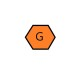I need to copy corresponding value of the cell

=IFERROR(IF(SEARCH(“*tain*”,A2,1),”Cell.Value(A2)”),”No”)
I need to copy the whole value of A2 in the cell.

15.Will this work in rows instead of searching in column?

16.Hello.

I am trying to figure out a formula which will help me do the following: in a certain cell i would like to search a keyword only in the first 4 characters. if the keyword is found in those 4 characters, the formula will return the cell without the 4 characters.

17.Hello,

I have some text in one cell like (ACC_MS_21x5_INC-Rsln-P3-ERP-EBS) I put your formula =IFERROR(IF(SEARCH("P3",A1,1),”P3”),”No”) according to my need, Here I want If the cell text contains P3 then I need to put value P3 in another column. But it throwing an error. Could you please let me know issue?

•It should work. If it is returning #NAME error then Try replacing ” with " in formula. It will work.
=IFERROR(IF(SEARCH("P3",A1,1),"P3"),"No")

18.Hi Guys,

In reference to the above example where the word "tain" is to be displayed, I have a list of 50-100 keywords, as in apple, orange, kiwi...etc 50-100 words.

If these keywords are found in a specific column then that keyword should be displayed in another column. Also, this list is subject to change by users as input.

I guess this can be resolved using a VBA.

19.Stephanie Rivera

I am trying to do this in Google Sheets and it's not working. Normally they are pretty much the same with their formulas.

=IF(ISNUMBER(SEARCH(“T*”,A9,1))=TRUE,”Trial”,IF(ISNUMBER(SEARCH(“C*”,A9,1))=TRUE,”MC”))

My question is, why are you putting the 1 in there?
And also why do you think mine isn't working?
If cell A9 starts with letter T, I want the other cell to say "Trial"
If cell A9 starts with letter C, I want the other cell to say "MC"

20.trying to search for 3 key words within text and return the found word back to another cell...using this formula.

=IFERROR(IF(SEARCH("*FIELD*",AU11,1),"FIELD"),"(IF(SEARCH("*FCS*",AU11,1),"FCS"),"(IF(SEARCH("*CPP*",AU11,1),"CPP"),"?")

Excel won't allow it, what am I missing?

21.What if I want to find multiple values in the original cell? Such as two years listed at different points in the text string...

22.I am trying o use the formula across sheet in a workbook but with no luck !!!
If in the sheet ISS Server Column I I have Microsoft windows server 2003 write in the sheet sheet2 the column A to I from ISS Server..

=IF(ISNUMBER(SEARCH("*Microsoft Windows Server 2003*",'ISS Servers'!H2)),'ISS Servers!H2'," ")
any idea?

Thanks,
Dom

23.Also in nested formula, I have 4 categories. If there is a prefix PSERI, it should be called Maintenance, prefix of PSI will be called Sales and SER & S-INV will be called Unposted. Is this possible and how?

24.How would I do this in a nested formula? Say if I had three categories
not 2. ie if search tain then call it 'tain', if search tra then call it tra if none found call it no

IFERROR(IF(SEARCH(“*tain*”,A2,1),”tain”),”(IF(SEARCH(“*tra*”,A2,1),”tra”),”No”)

•Hi Craig,

You can use below formula to solve your problem:-

=IF(ISNUMBER(SEARCH("tain",J7,1))=TRUE,"tain",IF(ISNUMBER(SEARCH("tra",J7,1))=TRUE,"tra","No"))

Thanks

•How would you use this formula to search a range of fields - and if any of the fields contained specified text you would return XYZ text result?

•What if you have 100's of criteria which bring up multiple regions. Would you have to do a very large formula or is there a quicker way?

•I was trying to get the formula to return three different statements but it hangs up at =IF(ISNUMBER(SEARCH(“tain”,J7,1))=TRUE,”tain”,IF(ISNUMBER(SEARCH(“tra”,J7,1))=TRUE,”tra”,”No”)) the second IF(ISNUMBER and thinks that the rest is for untrue so it just returns #NAME?. Also I would like it to return a blank cell if there is nothing in the first cell.

•Hi Eric,
You don't need True in formula. Try this formula.
=IF(ISNUMBER(SEARCH("tain",J7,1)),"tain",IF(ISNUMBER(SEARCH("tra",J7,1)),"tra",IF(ISBLANK(J7),"","No")))

•i need a formula to find out if column/row e2 contains the same text from column p2 or q2

25.I copied the formula: =IFERROR(IF(SEARCH(“*tain*”,A2,1),”tain”),”No”)
into cell b3, and after hitting return, got an Excel error "The formula you typed contains an error."

•Hi Mike,

After copy the formula in Excel please overwrite the quotation mark (") with quotation mark (") then press enter. you will get the appropriate result.

•Julie, Mike's cousin

May I suggest that it would be helpful to explain why you advise this? The computer converted the quotation marks in your answer to "smart" quotes, so I believe the spirit of the answer was lost. In the formula on this page, the quotation marks are word processed quotations rather than the quotation mark as typed by the keyboard. Excel formulas require the regular quotation mark rather than the nice looking quotation marks. Thanks!

•Not wishing to be the only one to state the obvious, are you sure this is correct?
There is no data in cell rage A2 to A7 in the examples above.
The data is in cells A5 to A10!

Terms and Conditions of use

The applications/code on this site are distributed as is and without warranties or liability. In no event shall the owner of the copyrights, or the authors of the applications/code be liable for any loss of profit, any problems or any damage resulting from the use or evaluation of the applications/code.